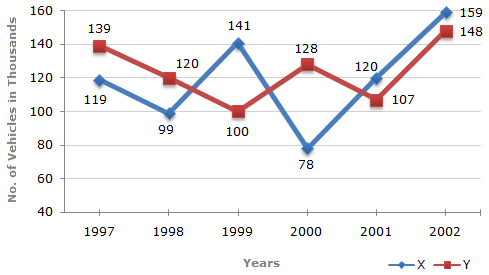### Line Chart - 2

Study the following line graph and answer the questions based on it.

Number of Vehicles Manufactured by Two companies ove the Years (Number in Thousands)1.  What is the difference between the total productions of the two Companies in the given years ?

 A. 19000 B. 22000 C. 26000 D. 28000 E. 29000
2.  What is the difference between the number of vehicles manufactured by Company Y in 2000 and 2001 ?

 A. 50000 B. 42000 C. 33000 D. 21000 E. 13000
3.  The production of Company Y in 2000 was approximately what percent of the production of Company X in the same year ?

 A. 173 B. 164 C. 132 D. 97 E. 61
4.  In which of the following years, the difference between the productions of Companies X and Y was the maximum among the given years ?

 A. 1997 B. 1998 C. 1999 D. 2000 E. 2001
5.  What is the average numbers of vehicles manufactured by Company X over the given period ? (rounded off to nearest integer)

 A. 119333 B. 113666 C. 112778 D. 111223 E. None of these